# What is the Naive Bayesian Classification Algorithm

## Puzzle ITC - Changing IT for the better

November 21, 2016

Blog post series part 2: On the trail of logic

In the first blog post I introduced a practical use case for machine learning and outlined a rough solution. In this second blog post I would like to take a closer look at the algorithms used. Therefore, this is probably the driest blog post we have ever published here, but to understand how the described machine learning approach works, we have to dive a little into the math.When looking for a solution for allocating budget items, I received the tip that a Bayesian classifier is a possible approach. Over time, through tests and various experiments, I have found out for the problem of budget item allocation described in the first blog post that the use of a Bayesian network brings the best results. What was also exciting in the search for a solution was the fact that such a classification gives better results than much more complex approaches (such as neural networks or the nearest neighbor algorithm). It should already be clear at this point that there is no simple solution to the subject of machine learning. The solution shown here is also specific for this application.

To explain the mathematical approach behind budget allocation, let me first define and explain a few terms;

### Definitions of terms

attribute

Attributes correspond to properties of an object. For example, a “Payment” object can have an “Amount” attribute. Every attribute has a set of values ​​that it can assume.

Attribute value

Attribute values ​​(also called characteristics) are defined units that belong to an attribute. These can be numerical values ​​or a fixed set of values, e.g.class

The class, also known as the class attribute, is the attribute you are looking for. Each classification tries to search for a class or to calculate a class value on the basis of a set of attributes.

Class value

A class value corresponds to a value that the class can assume, e.g .:object

An object has properties, e.g. a payment.

example

Once classified, objects are referred to as “examples”.

Training data

Training data is the set of possible examples that can be used as references for future classifications.

Training set

The training data that is used as a learning basis for a classification is called a training set or test data.

Since a set of attributes can also be viewed as the coordinate of a vector in n-dimensional space, we also speak of property vectors. Thus, an example or object of the training data corresponds to a vector or a feature vector in space.

object

Is referred to in space as a property vector or feature vector.

Training data

Now correspond to a set of classified property vectors.

In the case of budget item allocation, a payment would be an object for which we create a property vector. This is assigned to a class attribute by means of classification, in the case of budget item allocation to a budget item.

For the use of a classifier as an allocation basis for the selection of budget items, the use of a self-learning automatic classifier made sense to me. The aim of classifying learning is to predict the characteristics of a certain attribute, the values ​​of which are only known for part of the data. In other words: We do not know the class for all the properties of a payment. Examples for which we know the class are called classified examples. Accordingly, we do not know the class of unclassified examples.

Classifying learning consists of two steps. The first step consists of building or training a classifier, with which we search for and assign classes for unclassified property vectors in a second step. This assignment is called classification.

### Bayesian classification

A Bayesian classifier assigns each object to the class to which it belongs with the greatest probability or for which the classification results in the least costs. It is a mathematical function that assigns each point of a feature space to a class. The Bayesian classifier uses a cost measure that assigns costs to each possible classification. The Bayesian classifier tries to minimize these costs and to find the optimal allocation. In the simplest case, a cost measure is assumed which only incurs costs if there is a wrong decision. The aim of the classifier is therefore to keep costs as low as possible. However, the prerequisite for the creation and assignment of a cost measure is that it is known with what probability a point in the feature space belongs to a certain class. However, since this probability is not known in a practical application, it is estimated. One starts from a probability distribution (usually a normal distribution) and tries to estimate its parameters.
The Bayesian classifier applies the mathematical principles of Bayes' theorem.

### Bayes' theorem

Bayes 'theorem, or Bayes' theorem, describes how to calculate with conditional probabilities. Bayes' theorem for two random events A and B is as follows:The conditional probabilities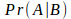and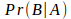let's call them a posteriori Probabilities. In contrast, we denote the probabilities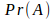and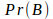as a priori Probabilities.

Bayes' theorem can be illustrated with a simple urn example. In two urns A and B there are red and white balls. In A there are seven red balls and three white balls, in B one red and nine white balls. Now any ball is drawn from A or B. Whether it is drawn from A or B, be a priori equally likely. The ball drawn is red. We are looking for the probability that this ball comes from urn A. Since the choice of the urn is equally likely, the result is:Since the number of balls and their color in both urns is known, we get for urn A:and for urn B:this results in a probability of pulling a red ball:Using Bayes' theorem, we now get the probability that a red ball from urn A comes from:### Naive Bayes classification

Bayes' theorem enables the probability of a class to be estimated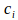for a given classification example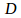. This probability can be used to classify the feature vector by predicting the class for which the highest probability has been estimated. We are at the a posteriori Probabilities for a classunder the occurrence of the vector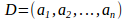Interested. Let's sitandinto Bayes' theorem, we get the following calculation approach:In the case of the naive Bayesian classification, the classification problem can be expressed as the probability that a payment, given by the feature vector, the classis to be assigned to be described. So for all class attributes the conditional probability becomes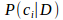calculated.

If allare known, a payment with feature vectorin the classbe classified for whichassumes the greatest value. Since only nominal attributes are used in the budget item assignment, I will limit myself to the calculation with nominal values. Since rarely allare known, the following approach is followed:in which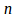the number of training sets and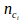the number of training sets in the classhave been assigned. The absolute frequency of the classis divided by the number of training sets. To determine this, all training sets are used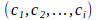go through andis increased accordingly. The probability that exactly the vector we are looking for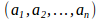is already in the training set, is relatively small or does not exist. The lack of vectors would be useful in estimating the probabilities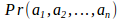lead to very small, insufficiently meaningful values. Therefore becomes a naive Assumption made by assuming all attributes to be independent. This results in a possibility of estimating the desired, conditional probabilities. Because of this independence assumption, this becomes Bayesian classifier as more naiveBayesian classifier designated.The probability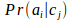can be estimated independently of the other attributes. For this purpose, the relative frequency of the vectors for which the attribute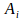the value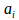and the class attribute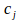is, by the relative frequency of the classdivided.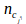corresponds to the number of training sets with a class attributeand attribute valuefor the attribute. This can already be used to determine the number of training sets with class attribute when running through the training dataTo be defined. However, there is a problem with this: if the absolute frequencyof an attribute valuethe classIs 0, this also applies to the probability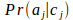and thus also for the product of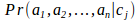. As a result, the remaining probabilities become irrelevant. One solution to this problem is that m-estimate. This is based on an a priori estimate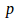the probabilityout.

m-estimate:m stands for the equivalent sample size. This is a constant that determines how strongis weighted relative to the trained data. The name equivalent samplesize comes through a possible interpretation as an extension of theAttributes in the classesaround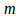virtual attributes, whichare distributed accordingly. Using the m-estimate the following formula results for the naive Bayesian classification:### Bayesian networks

In contrast to the naive Bayesian approach, the application of a classification using Bayesian networks does not assume that the attributes are independent. Dependencies between individual attributes can be defined in the form of a directed acyclic graph (DAG), with nodes representing features and their edges representing dependencies between features. The naive Bayesian classifier can also be viewed as a special case of a Bayesian network classifier in that a star-shaped Bayesian network is created in which all attributes only depend on the class.Each node can have a probability value from 0 to 1. The probabilities of the successor nodes are calculated with these probabilities. A table with conditional probabilities is calculated for each node. If a node has only one predecessor node, this can be a simple conditional probability.

The table of conditional probabilities contains direct predecessor features for each possible combination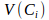the conditional probability for the characteristic valuesas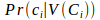. This allows withthe probability for a certain path of characteristic values ​​can be calculated. One or more nodes of the graph are selected as target nodes for the classification. These correspond to the group according to which the objects are to be classified. In order to classify an object, the probabilities of the paths to the selected target nodes are compared and the target node with the highest probability is selected.

### Back to the practice

Since I do not want to implement the complete algorithm in an application, I decided to use WEKA, a Java library that provides a large number of algorithms ready-made. The principle of the calculation remains the same. For each feature of my payment, the probability for each possible budget item is calculated. In the end, WEKA gives me a list of my budget items and the respective percentage allocation probability. I only have to select the budget item with the highest value and get a relatively high hit rate. Of course, everything can still be optimized. For example, through the use of a Bayesian network, in which dependencies are defined under the payment characteristics. For example, I can define that the type of purchase is directly dependent on the day of the week. Since I usually do bulk shopping and clothing purchases on Saturdays, this already results in an improvement.

How I integrated Weka in my solution and how to work with the API of the library I will explain in the next blog post.

Read the third part of the blog post series here.

Read the first part of the blog post series here.